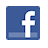Games
Problems
Go Pro!

Testing for Zero Values

Reference > Science > Technology > Beginner Programming Tips

Suppose you have 3 variables: X, Y and Z. And you want to find out if any of them are equal to zero. Here's how you might do it?

Dim MyBoolean As Boolean
Dim X As Integer
Dim Y As Integer
Dim Z As Integer

'Check to see if any of X, Y or Z equal 0
MyBoolean = False
If X = 0 Then
If Y = 0 Then
If Z = 0 Then
MyBoolean = True
End If
End If
End If

Is that the quickest and easiest way to do it? No. Here's another way:

Dim MyBoolean As Boolean
Dim X As Integer
Dim Y As Integer
Dim Z As Integer

'Check to see if any of X, Y or Z equal 0
MyBoolean = (X = 0) Or (Y = 0) Or (Z = 0)

Much shorter, much simpler. Is there a shorter way still of doing it?

Yes, there is. But before I give it to you, I have to warn you, there are some warnings and disclaimers that go with this method. So be sure to read the paragraphs after the code to find out what the disclaimers are.

Here's the code.

Dim MyBoolean As Boolean
Dim X As Integer
Dim Y As Integer
Dim Z As Integer

'Check to see if any of X, Y or Z equal 0
MyBoolean = X * Y * Z

Why does this work? Because if any of X, Y, or Z is equal to 0, the whole equation is equal to zero, and we already know that the computer treats zero as a boolean False! If none of them are zero, then MyBoolean turns out to be a non-zero number, and the computer considers any non-zero number to have a truth value of True

Now, what about those disclaimers? The first disclaimer is this: There is no guarantee that one method is quicker than another to execute. That is largely dependent on the variable types you are using. When I set X, Y, and Z to Long variables, the multiplication method was actually about 4 times as fast. However, when I set them to Double variable types, there was no signicant speed difference between the methods.

The second disclaimer is this: you should not do this unless you are sure that your variables X, Y, and Z are small numbers. What happens if X, Y, and Z all equal 100? When you multiply them as Integers, you get an overflow error!

And the third disclaimer is, this method is not asreadable. In other words, when you come back to this line of code after five months, you're going to look at it and say "What in the WORLD does that line do?"

But even with all the disclaimers, and even though you probably won't ever do it in an honest-to-goodness program, it's still kind of a fun trick.

One more cute little trick. Suppose you wanted to check to find out if X = 0 or Y = 1. You could do it like this:

Dim MyBoolean As Boolean
Dim X As Integer
Dim Y As Integer

'Check to see if X = 0 or Y = 1
MyBoolean = X * (Y - 1)

And why do you suppose that works? Because if Y equals one then (Y - 1) equals zero!

Questions

1.
Write a line of code to determine if X = 0 or Y = 1 or Z = 2.
2.
Write a line of code to determine if X, Y, or Z equal 5.
3.
Now write those same lines of code without using the "mutliplication trick."Assign this reference pageCounting Truth ValuesTwo Dimensions, One Dimension

Featured Games on This Site

Match color, font, and letter in this strategy game
Trap all the dots in this problem-solving puzzle

Blogs on This SiteReviews and book lists - books we love!The site administrator fields questions from visitors.Like us on Facebook to get updates about new resources